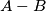### IMO Shortlist 2006 problem C7

Kvaliteta:
Avg: 0,0
Težina:
Avg: 9,0
Consider a convex polyhaedron without parallel edges and without an edge parallel to any face other than the two faces adjacent to it. Call a pair of points of the polyhaedron antipodal if there exist two parallel planes passing through these points and such that the polyhaedron is contained between these planes. Let$A$ be the number of antipodal pairs of vertices, and let$B$ be the number of antipodal pairs of midpoint edges. Determine the difference$A-B$ in terms of the numbers of vertices, edges, and faces.
Izvor: Međunarodna matematička olimpijada, shortlist 2006